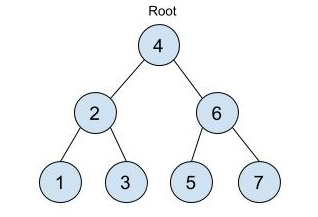New update is available. Click here to update.

# House Robber III

Posted: 25 Mar, 2021
Difficulty: Moderate

## PROBLEM STATEMENT

#### Input Format:

``````The first line of input contains an integer 'T' representing the number of test cases. Then the test cases follow.

The first line of each test case contains a fun number assigned to each ride in the level order form. The line consists of values of nodes separated by a single space. In case a node is null, we take -1 in its place.

For example, the input for the tree depicted in the below image would be :
````````````4
2 6
1 3 5 7
-1 -1 -1 -1 -1 -1
``````

#### Explanation:

``````Level 1 :
The root node of the tree is 4

Level 2 :
Left child of 4 = 2
Right child of 4 = 6

Level 3 :
Left child of 2 = 1
Right child of 2 = 3
Left child of 6 = 5
Right child of 6 = 7

Level 4 :
Left child of 1 = null (-1)
Right child of 1 = null (-1)
Left child of 3 = null (-1)
Right child of 3 = null (-1)
Left child of 5 = null (-1)
Right child of 5 = null (-1)
Left child of 7 = null (-1)
Right child of 7 = null (-1)

The first not-null node(of the previous level) is treated as the parent of the first two nodes of the current level. The second not-null node (of the previous level) is treated as the parent node for the next two nodes of the current level and so on.

The input ends when all nodes at the last level are null(-1).
``````
##### Note:
``````The above format was just to provide clarity on how the input is formed for a given tree.

The sequence will be put together in a single line separated by a single space. Hence, for the above-depicted tree, the input will be given as:

4 2 6 1 3 5 7 -1 -1 -1 -1 -1 -1
``````

#### Output Format:

``````For each test case, print the maximum fun that ninja can get from the amusement park.

The output for each test case is printed in a separate line.
``````

#### Constraints:

``````1 <= T <= 5
1 <= N <= 5* 10^3
1 <= DATA <= 10^4

Time limit: 1 sec
``````
SIMILAR PROBLEMS

Capturing Grid

Posted: 14 Sep, 2022
Difficulty: Moderate

Rotting Oranges

Posted: 15 Sep, 2022
Difficulty: Moderate

Distance to a Cycle in Undirected Graph

Posted: 7 Nov, 2022
Difficulty: Moderate

Randomly Sorted

Posted: 13 Nov, 2022
Difficulty: Moderate

8-Queen Problem

Posted: 19 Dec, 2022
Difficulty: Easy